# CBSE Sample Papers for Class 12 Maths UnSolved 2016 Set 9

0
1107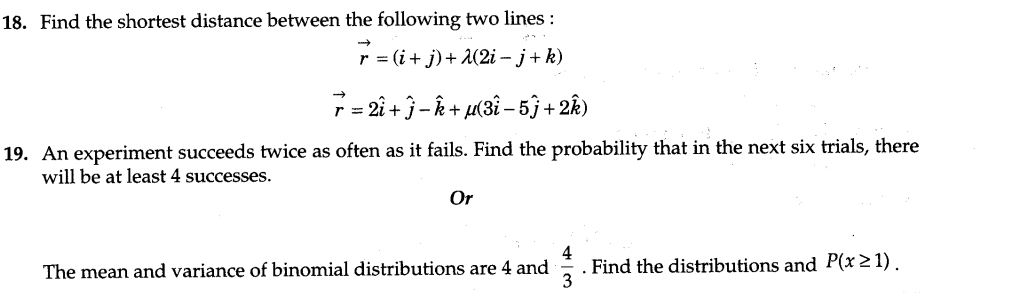SECTION — A

Question numbers 1 to 6 carry 1 mark each.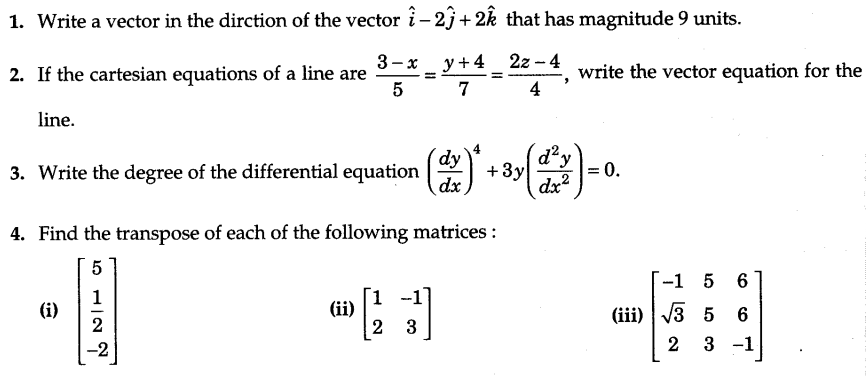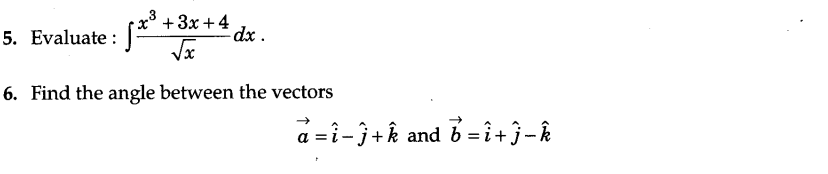SECTION — B

Question numbers 7 to 19 carry 4 marks each.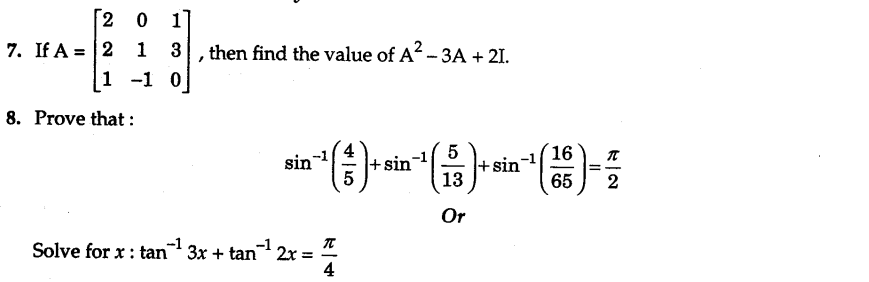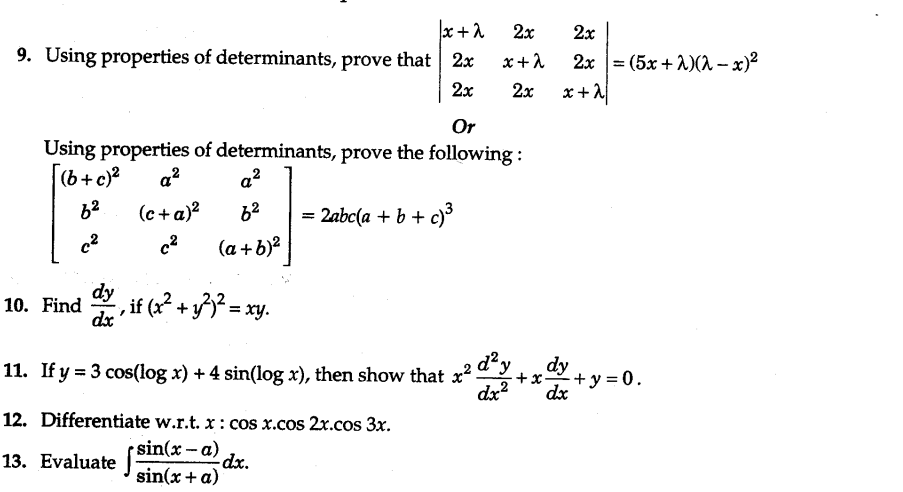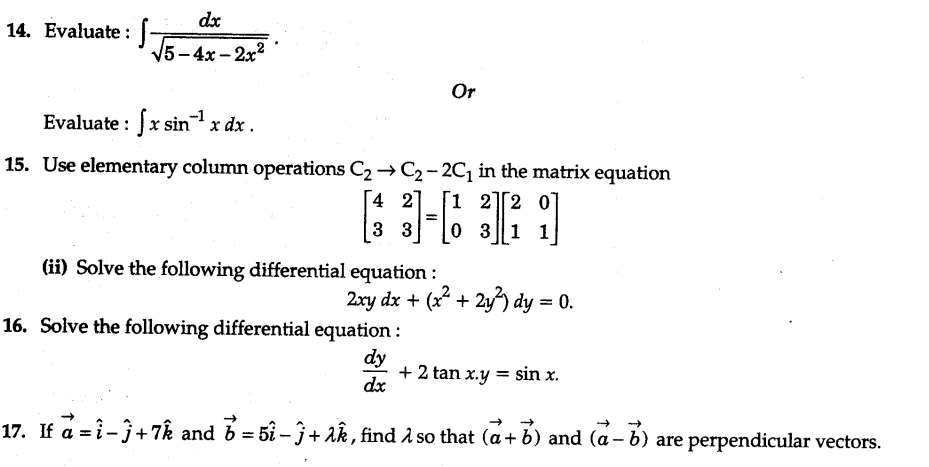SECTION — C

Question numbers 20 to 26 carry 6 marks each.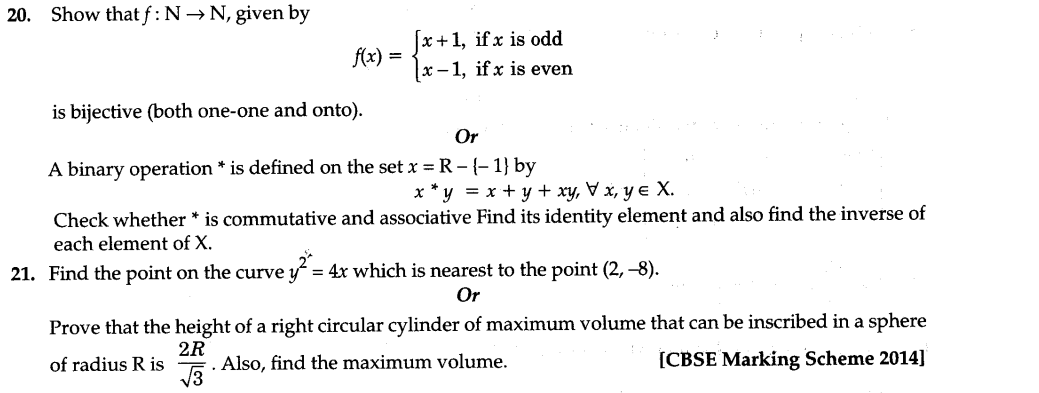22.Find the area of the region included between the parabola 4y = 3x and the line 3x – 2y + 12 = 0.

23.Find the particular solution of the differential equation (x – sin y)dy = (tan y)dx = 0, given that y = 0 when x = 0.

24.The points A(4, 5,10), B(2, 3, 4) and C(l, 2, – 1) are three vertices of a parallelogram ABCD. Find the vector equations of the sides AB and BC and also find the coordinates of point D.

25.A toy company manufactures two types of dolls, A and B. Market tests and available resources have indicated that the combined production level should not exceed 1200 dolls per week and the demand for dolls of type B is atmost half of that for dolls of type A. Further, the production level of dolls of type A can exceed three times the production of dolls of other type by utmost 600 units. If the company makes profit of Rs 12 and Rs 16 per doll respectively on dolls A and B, how many of each should be produced weekly in order to maximize the profit ?

26.An insurance company issued 3000 scooters, 4000 cars and 5000 trucks. The probabilities of an accident involving a scooter, a car and a truck are 0.02, 0.03 and 0.04 respectively. One of the insured vehicle meets with an accident. Find the probability that (i) it is a scooter, (ii) it is a car, (iii) it is a truck.